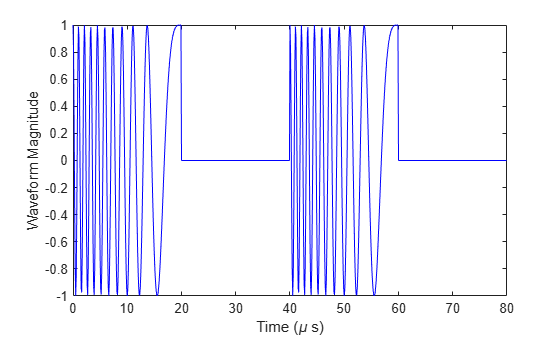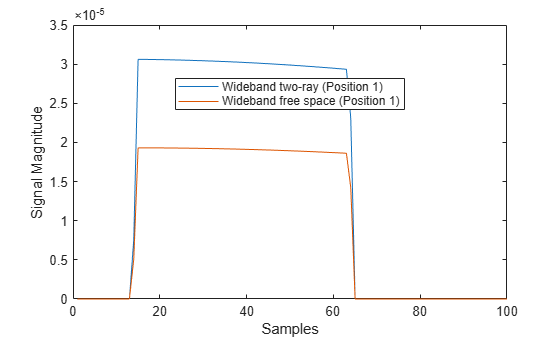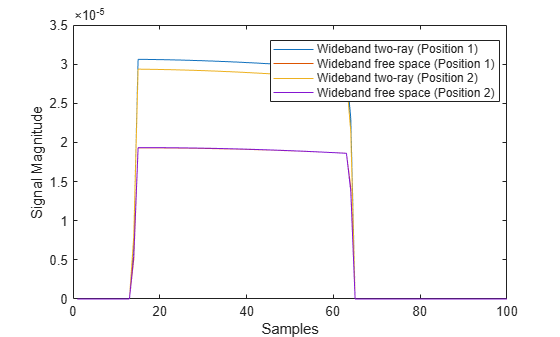# step

System object: widebandTwoRayChannel

Propagate wideband signal from point to point using two-ray channel model

## Syntax

prop_sig = step(channel,sig,origin_pos,dest_pos,origin_vel,dest_vel) 

## Description

Note

Alternatively, instead of using the step method to perform the operation defined by the System object™, you can call the object with arguments, as if it were a function. For example, y = step(obj,x) and y = obj(x) perform equivalent operations.

prop_sig = step(channel,sig,origin_pos,dest_pos,origin_vel,dest_vel) returns the resulting signal, prop_sig, when a wideband signal, sig, propagates through a two-ray channel from the origin_pos position to the dest_pos position. Either the origin_pos or dest_pos arguments can have multiple points but you cannot specify both as having multiple points. Specify the velocity of the signal origin in origin_vel and the velocity of the signal destination in dest_vel. The dimensions of origin_vel and dest_vel must agree with the dimensions of origin_pos and dest_pos, respectively.

In the two-ray environment, two signal paths connect every signal origin and destination pair. For N signal origins (or N signal destinations), there are 2N paths. The signals for each origin-destination pair do not have to be identical. The signals along the two paths for any source-destination pair can have different amplitudes or phases.

The CombinedRaysOutput property controls whether the two signals at the destination are kept separate or combined. Combined means that the signals at the source propagate separately along the two paths but are coherently summed at the destination into a single quantity. Separatemeans that the two signals are not summed at the destination. To use the combined option, set CombinedRaysOutput to true. To use the separate option, set CombinedRaysOutput to false. The combined option is convenient when the difference between the sensor or array gains in the directions of the two paths is not significant.

Note

The object performs an initialization the first time the object is executed. This initialization locks nontunable properties and input specifications, such as dimensions, complexity, and data type of the input data. If you change a nontunable property or an input specification, the System object issues an error. To change nontunable properties or inputs, you must first call the release method to unlock the object.

## Input Arguments

expand all

Wideband two-ray channel, specified as a System object.

Example: widebandTwoRayChannel

Electromagnetic fields propagated through a two-ray channel can be polarized or nonpolarized. For nonpolarized fields, such as an acoustic field, the propagating signal field, sig, is a vector or matrix. When the fields are polarized, sig is an array of structures. Every structure element contains an array of electric field vectors in Cartesian form.

• Specify wideband nonpolarized scalar signals as a

• M-by-N complex-valued matrix. The same signal is propagated along both the line-of-sight path and the reflected path.

• M-by-2N complex-valued matrix. Each adjacent pair of columns represents a different channel. Within each pair, the first column represents the signal propagated along the line-of-sight path and the second column represents the signal propagated along the reflected path.

• Specify wideband polarized signals as a

• 1-by-N struct array containing complex-valued fields. Each struct element contains an M-by-1 column vector of electromagnetic field components (sig.X,sig.Y,sig.Z). The same signal is propagated along both the line-of-sight path and the reflected path.

• 1-by-2N struct array containing complex-valued fields. Each pair of array columns represents a different source-receiver channel. The first column of the pair represents the signal along the line-of-sight path and the second column represents the signal along the reflected path. Each structure element contains an M-by-1 column vector of electromagnetic field components (sig.X,sig.Y,sig.Z).

For nonpolarized fields, the quantity M is the number of samples of the signal and N is the number of two-ray channels. Each channel corresponds to a source-destination pair.

The size of the first dimension of the input matrix can vary to simulate a changing signal length. A size change can occur, for example, in the case of a pulse waveform with variable pulse repetition frequency.

For polarized fields, the struct element contains three M-by-1 complex-valued column vectors, sig.X, sig.Y, and sig.Z. These vectors represent the x, y, and z Cartesian components of the polarized signal.

The size of the first dimension of the matrix fields within the struct can vary to simulate a changing signal length such as a pulse waveform with variable pulse repetition frequency.

Example: [1,1;j,1;0.5,0]

Data Types: double
Complex Number Support: Yes

Origin of the signal or signals, specified as a 3-by-1 real-valued column vector or 3-by-N real-valued matrix. The quantity N is the number of two-ray channels. If origin_pos is a column vector, it takes the form [x;y;z]. If origin_pos is a matrix, each column specifies a different signal origin and has the form [x;y;z]. Position units are in meters.

You cannot specify both origin_pos and dest_pos as matrices. At least one must be a 3-by-1 column vector.

Example: [1000;100;500]

Data Types: double

Destination position of the signal or signals, specified as a 3-by-1 real-valued column vector or 3-by-N real-valued matrix. The quantity N is the number of two-ray channels propagating from or to N signal origins. If dest_pos is a 3-by-1 column vector, it takes the form [x;y;z]. If dest_pos is a matrix, each column specifies a different signal destination and takes the form [x;y;z] Position units are in meters.

You cannot specify both origin_pos and dest_pos as matrices. At least one must be a 3-by-1 column vector.

Example: [0;0;0]

Data Types: double

Velocity of signal origin, specified as a 3-by-1 real-valued column vector or 3-by-N real-valued matrix. The dimensions of origin_vel must match the dimensions of origin_pos. If origin_vel is a column vector, it takes the form [Vx;Vy;Vz]. If origin_vel is a 3-by-N matrix, each column specifies a different origin velocity and has the form [Vx;Vy;Vz]. Velocity units are in meters per second.

Example: [10;0;5]

Data Types: double

Velocity of signal destinations, specified as a 3-by-1 real-valued column vector or 3–by-N real-valued matrix. The dimensions of dest_vel must match the dimensions of dest_pos. If dest_vel is a column vector, it takes the form [Vx;Vy;Vz]. If dest_vel is a 3-by-N matrix, each column specifies a different destination velocity and has the form [Vx;Vy;Vz] Velocity units are in meters per second.

Example: [0;0;0]

Data Types: double

## Output Arguments

expand all

• Wideband nonpolarized scalar signal, returned as an:

• M-by-N complex-valued matrix. To return this format, set the CombinedRaysOutput property to true. Each matrix column contains the coherently combined signals from the line-of-sight path and the reflected path.

• M-by-2N complex-valued matrix. To return this format set the CombinedRaysOutput property to false. Alternate columns of the matrix contain the signals from the line-of-sight path and the reflected path.

• Wideband polarized scalar signal, returned as:

• 1-by-N struct array containing complex-valued fields. To return this format, set the CombinedRaysOutput property to true. Each column of the array contains the coherently combined signals from the line-of-sight path and the reflected path. Each structure element contains the electromagnetic field vector (prop_sig.X,prop_sig.Y,prop_sig.Z).

• 1-by-2N struct array containing complex-valued fields. To return this format, set the CombinedRaysOutput property to false. Alternate columns contains the signals from the line-of-sight path and the reflected path. Each structure element contains the electromagnetic field vector (prop_sig.X,prop_sig.Y,prop_sig.Z).

The output prop_sig contains signal samples arriving at the signal destination within the current input time frame. Sometimes it can take longer than the current time frame for the signal to propagate from the origin to the destination, the output may not contain all contributions from the input of the current time frame. In this case, the output does not need to contain all contributions from the input of the current time frame. The remaining output appears in the next call to step.

## Examples

expand all

This example illustrates the two-ray propagation of a wideband signal, showing how the signals from the line-of-sight path and reflected path arrive at the receiver at different times.

Note: You can replace each call to the function with the equivalent step syntax. For example, replace myObject(x) with step(myObject,x).

Create and Plot Transmitted Waveform

Create a nonpolarized electromagnetic field consisting of two linear FM waveform pulses at a carrier frequency of 100 MHz. Assume the pulse width is 20 μs and the sampling rate is 10 MHz. The bandwidth of the pulse is 1 MHz. Assume a 50% duty cycle so that the pulse width is one-half the pulse repetition interval. Create a two-pulse wave train. Set the GroundReflectionCoefficient to –0.9 to model strong ground reflectivity. Propagate the field from a stationary source to a stationary receiver. The vertical separation of the source and receiver is approximately 10 km.

c = physconst('LightSpeed'); fs = 10e6; pw = 20e-6; pri = 2*pw; PRF = 1/pri; fc = 100e6; lambda = c/fc; bw = 1e6; waveform = phased.LinearFMWaveform('SampleRate',fs,'PulseWidth',pw,... 'PRF',PRF,'OutputFormat','Pulses','NumPulses',2,'SweepBandwidth',bw,... 'SweepDirection','Down','Envelope','Rectangular','SweepInterval',... 'Positive'); wav = waveform(); n = size(wav,1); plot([0:(n-1)]/fs*1e6,real(wav),'b') xlabel('Time (\mu s)') ylabel('Waveform Magnitude')Specify the Location of Source and Receiver

Place the source and receiver about 1 km apart horizontally and approximately 5 km apart vertically.

pos1 = [0;0;100]; pos2 = [1e3;0;5.0e3]; vel1 = [0;0;0]; vel2 = [0;0;0];

Create a Wideband Two-Ray Channel System Object

Create a two-ray propagation channel System object™ and propagate the signal along both the line-of-sight and reflected ray paths. The same signal is propagated along both paths.

channel = widebandTwoRayChannel('SampleRate',fs,... 'GroundReflectionCoefficient',-0.9,'OperatingFrequency',fc,... 'CombinedRaysOutput',false); prop_signal = channel([wav,wav],pos1,pos2,vel1,vel2); [rng2,angs] = rangeangle(pos2,pos1,'two-ray');

Calculate time delays in μs.

tm = rng2/c*1e6; disp(tm)
 16.6815 17.3357 

Display the calculated propagation paths azimuth and elevation angles in degrees.

disp(angs)
 0 0 78.4654 -78.9063 

Plot the Propagated Signals

1. Plot the real part of the signal propagated along the line-of-sight path.

2. Plot the real part of the signal propagated along the reflected path.

3. Plot the real part of the coherent sum of the two signals.

n = size(prop_signal,1); delay = [0:(n-1)]/fs*1e6; subplot(3,1,1) plot(delay,real([prop_signal(:,1)]),'b') grid xlabel('Time (\mu sec)') ylabel('Real Part') title('Direct Path') subplot(3,1,2) plot(delay,real([prop_signal(:,2)]),'b') grid xlabel('Time (\mu sec)') ylabel('Real Part') title('Reflected Path') subplot(3,1,3) plot(delay,real([prop_signal(:,1) + prop_signal(:,2)]),'b') grid xlabel('Time (\mu sec)') ylabel('Real Part') title('Combined Paths')The delay of the reflected path signal agrees with the predicted delay. The magnitude of the coherently combined signal is less than either of the propagated signals. This result indicates that the two signals contain some interference.

Compute the result of propagating a wideband LFM signal in a two-ray environment from a radar 10 meters above the origin (0,0,10) to a target at (3000,2000,2000) meters. Assume that the radar and target are stationary and that the transmitting antenna is isotropic. Combine the signal from the two paths and compare the signal to a signal propagating in free space. The system operates at 300 MHz. Set the CombinedRaysOutput property to true to combine the direct path and reflected path signals when forming the output signal.

Note: This example runs only in R2016b or later. If you are using an earlier release, replace each call to the function with the equivalent step syntax. For example, replace myObject(x) with step(myObject,x).

Create a linear FM waveform.

fop = 300.0e6; fs = 1.0e6; waveform = phased.LinearFMWaveform(); x = waveform();

Specify the target position and velocity.

posTx = [0; 0; 10]; posTgt = [3000; 2000; 2000]; velTx = [0;0;0]; velTgt = [0;0;0];

Model the free space propagation.

fschannel = phased.WidebandFreeSpace('SampleRate',waveform.SampleRate); y_fs = fschannel(x,posTx,posTgt,velTx,velTgt);

Model two-ray propagation from the position of the radar to the target.

tworaychannel = widebandTwoRayChannel('SampleRate',waveform.SampleRate,... 'CombinedRaysOutput',true); y_tworay = tworaychannel(x,posTx,posTgt,velTx,velTgt); plot(abs([y_tworay y_fs])) legend('Wideband two-ray (Position 1)','Wideband free space (Position 1)',... 'Location','best') xlabel('Samples') ylabel('Signal Magnitude') hold onMove the radar by 10 meters horizontally to a second position.

posTx = posTx + [10;0;0]; y_fs = fschannel(x,posTx,posTgt,velTx,velTgt); y_tworay = tworaychannel(x,posTx,posTgt,velTx,velTgt); plot(abs([y_tworay y_fs])) legend('Wideband two-ray (Position 1)','Wideband free space (Position 1)',... 'Wideband two-ray (Position 2)','Wideband free space (Position 2)',... 'Location','best') hold offThe free-space propagation losses are the same for both the first and second positions of the radar. The two-ray losses are different due to the interference effect of the two-ray paths.

## Extended Capabilities

### C/C++ Code GenerationGenerate C and C++ code using MATLAB® Coder™.

Introduced in R2021a